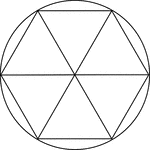### Regular Hexagon Inscribed In A Circle

Illustration of a regular hexagon inscribed in a circle. This can also be described as a circle circumscribed…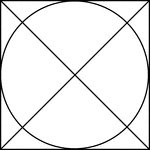### Square Circumscribed About A Circle

Illustration of a square, with diagonals drawn, circumscribed about a circle. This can also be described…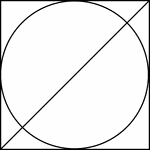### Square Circumscribed About A Circle

Illustration of a square, with 1 diagonals drawn, circumscribed about a circle. This can also be described…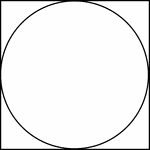### Square Circumscribed About A Circle

Illustration of a square circumscribed about a circle. This can also be described as a circle inscribed…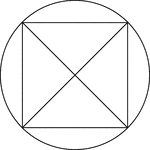### Square Inscribed In A Circle

Illustration of a square, with diagonals drawn, inscribed in a circle. This can also be described as…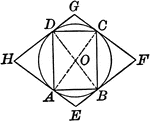### Circle With Inscribed and Circumscribed Quadrilaterals

Illustration showing a circle with an inscribed and circumscribed quadrilateral.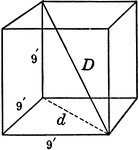### Cube 9 by 9 by 9 With Diagonals

9' by 9' by 9' Cube with diagonals labeled.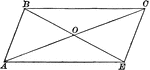### Parallelogram With Diagonals Bisecting Each Other

Illustration of a parallelogram with diagonals drawn to show they bisect each other.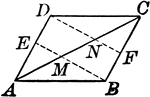### Parallelogram With Lines From opposite Vertices to Midpoints

Illustration to show that when lines from two opposite vertices of a parallelogram to the midpoints…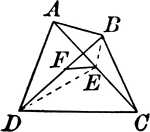### Quadrilateral With Diagonals and Midpoints Joined

Quadrilateral with intersecting diagonals and segment connecting midpoints.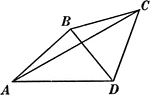Illustration of a quadrilateral with diagonals AC and BD.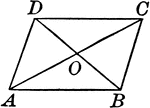Illustration to show that if the diagonals of a quadrilateral bisect each other, the figure is a parallelogram.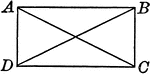### Rectangle With Diagonals

Illustration to show that if the diagonals of a parallelogram are equal, the figure is a rectangle.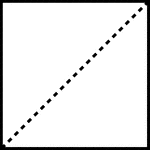### Divided Square

A square divided evenly by a dotted line, known as the diagonal.### Square With Diagonals and Lines Drawn

Illustration showing a square with diagonals, lines, and parallel lines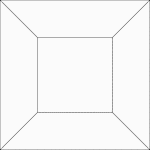### 2 Concentric Squares

Illustration of 2 concentric squares whose vertices are connected by line segments.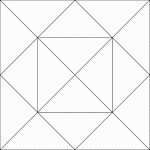### Squares Inscribed In Squares

Illustration of a square inscribed in a square that is inscribed in another square. Each successive…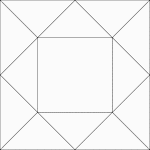### Squares Inscribed In Squares

Illustration of a square inscribed in a square that is inscribed in another square. Each successive…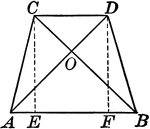### Isosceles Trapezoid With Equal Diagonals

Illustrations of an isosceles trapezoid with equal diagonals drawn.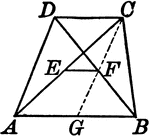### Trapezoid With Lines Joining the Midpoints of the Diagonals

Illustrations of a trapezoid with a line joining the midpoints of the diagonals.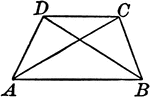### Trapezoid With Diagonals

Illustrations of a trapezoid with diagonals drawn.